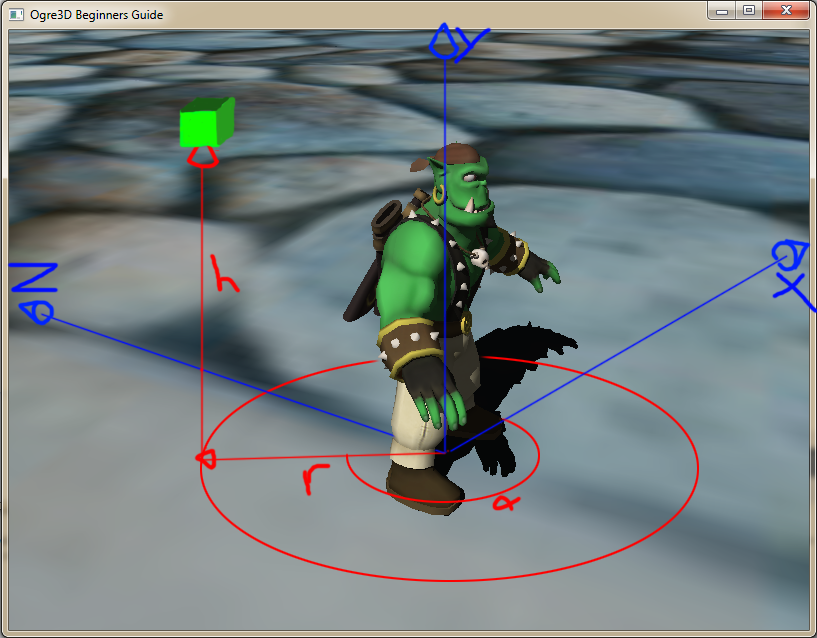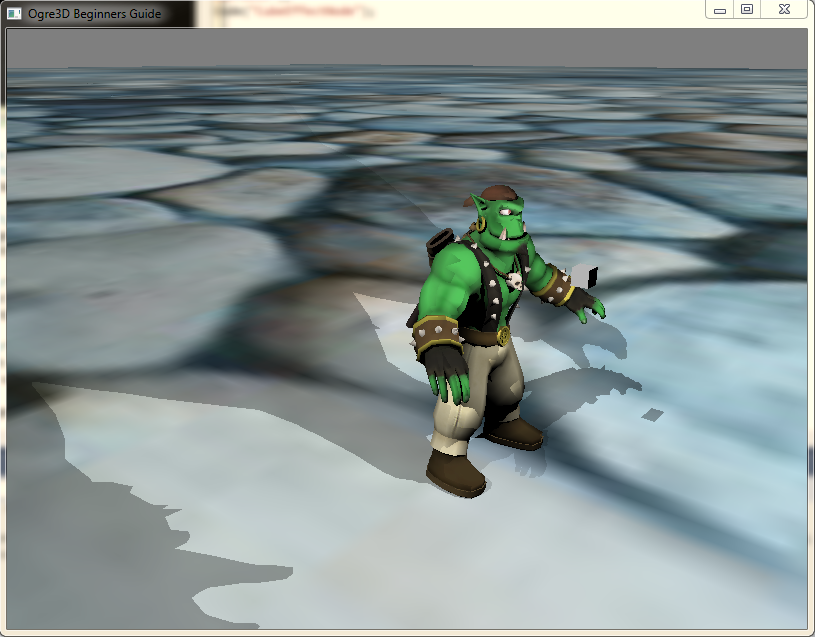#Center for Analysis and Design of Intelligent Agents

### Site Tools

public:t-gede-15-1:lab3

# LAB3: Applying Math

This lab is to some extent based on Chapter 4 in the textbook, in particular sections 4.2.1 “Points and Cartesian Coordinates” and 4.2.4 “Vector Operations”.

The main focus here is to learn how we can use other coordinate systems to help us generate effects or behaviour that would be unintuitive to do with Cartesian coordinates.

## Goal

There are two main parts to this lab. In the first part, which has you thinking about coordinate systems, you create an animation effect that spins an object along a cylindrical path. In the second part, which focuses on vector operations, you use a simple dot product to find which direction you are facing relative to another object.

## Preperation

1. Create a new Project with the instructions from lab 1 or 2.
2. Make sure you have the completed Lab2 project (the optional steps are not necessary)
3. `OgreMath.h` included in your source file to access the `Ogre::Math::Cos()` and `Ogre::Math::Sin()` functions

## Lab Project

Follow these steps to complete the lab project:

1. Attach an Object to the Ogre You should create a new movable entity that will be attached to the Ogre entity (from Lab2) wherever it goes. Essentially this new entity, that we will call “The Cube”, will exist in the coordinate system of the Ogre model. To do this, you need to create a new `SceneNode` as a child of the Ogre's scene node, and attach the Cube to that node. This means that all transformations applied to the Ogre's scene node will be passed down into the Cube's scene node as well. So, do the following:
1. Add a member variable to your application class to hold the old Ogre entity (e.g. “_myOgre”) and assign the entity to it when you create it in `createScene`
2. Add a member variable to your application class to hold the new Cube entity (e.g. “_myCube”).
3. In the `createScene` method, add the following (after you create the Ogre entity):
```_myCube = _sceneManager->createEntity("Cube.mesh");
Ogre::SceneNode* cubenode =_myOgre->getParentSceneNode()->createChildSceneNode();
cubenode->attachObject(_myCube);```
4. You need to scale and then translate (position) the new Cube so that you can see it next to the Ogre:
```cubenode->scale(0.01, 0.01, 0.01);    //Try different values
cubenode->setPosition(2.0, 0.0, 0.0); //Notice that this is relative to the Ogre's model origin```
2. Attach a Light to the Cube You can make it look like the Cube is a source of light that lights up the Ogre and casts a shadow on the ground. Simply attach a point light source to the Cube's scene node:
```Ogre::Light* plight = _sceneManager->createLight("Light2");
plight->setType(Ogre::Light::LT_POINT);
cubenode->attachObject(plight);```
3. Create a Cylindrical Effect Class This is the meat of the project. You should create a new class called `CylindricalEffect` that moves an entity along a cylindric path at a given velocity. The class has the following constructor:
`CylindricalEffect(Ogre::Entity* entity, Ogre::Vector3 position, Ogre::Vector3 velocity)`
4. You should store each of the arguments as a private member variable in the class. The first argument `entity` is any movable entity in the scene that you want to apply the animation effect to. The second argument `position` is that entity's position (relative to it's parent) in Cylindrical Coordinates, that is, the vector consists of three numbers:
1. First number is the height of the cylindrical position
2. Second number is the radius of the cylindrical position
3. Third is the angle (in radians) of the cylindrical position
The third argument `velocity` is the velocity of the object along the three axes of the cylindrical coordinates, i.e. along the height axis, along the radius and the angular velocity along the circle.
5. Add a `GetCartesian()` Method If the class operates on cylindrical coordinates, you will always want to retrieve the corresponding cartesian coordinates for updating positions in the Ogre3D default cartesian coordinate system. Therefore you should add the following method which should return the class's position member variable converted to Cartesian coordinates `Ogre::Vector3 getCartesian() `

Looking at this artistically gorgeous picture (Only constructive criticism accepted, I am an aspiring artist), you can get an idea on what cylindrical coordinates are and how you could convert them to Cartesian coordinates.Lets say:
``` (height,radius,angle) = (h, r, α)
X coordinate = cosine of the angle times the radius
Y coordinate = height
Z Coordinate = sin(angle) times the radius```
6. Add an Update Method The heart of this effect is a calculation of a new position for the entity based on linear movement along the three axes of the cylindrical coordinate system (height, radius and angle). Since we are already representing the position cylindrically, all we have to do is to update the position by adding the velocity vector times the time that has passed since the last update:
```void update(float dt) {
_position = _position + dt*_velocity;
_entity->getParentSceneNode()->setPosition(getCartesian());
}```
7. Instantiate Effect and Update from FrameListener Now you have to create an instance of your effect, passing the Cube entity into it's constructor, giving it initial position and velocity values, and finally make sure to call this effect's update function in every frame, e.g. inside the FrameListener's `frameStarted` method (note that you'll have to pass a pointer to this effect into the FrameListener constructor so that it knows about it). Don't forget to delete your effect instance in the destructor of your application!
8. Make the cube move in a non linear path, Now the cube moves linearly upward rotating around Sinbad, make the cube move non linearly, explore the use of cosine function with time on the cubes radius, height or velocity for interesting results.

## Bonus points!

Bonus Pts!

1. Activate Only when Facing Ogre To know if an object is in front of the camera, you can apply a simple dot product test. Take the vector between the camera and the object (use vector subtraction) and call that V. Now take the dot product between V and the facing vector F of the camera (`Ogre::Vector3 F = _Cam→getDirection();`). If this dot product is greater than 0, you know the object is in front of the camera. Finally, why don't you also check to see if the object is relatively close to the camera (you can use the Ogre::Vector3::length() method). Now you can add this to your project such that the special effect only gets updated when these two checks are passed!2. Make the cube an object that is lying on the ground, when the Ogre passes over the cube, he will pick it up and the animation will play the cube circling the Ogre like he just picked up a power-up.
Wouldn't it be would be fun to implement it with a random number of randomly placed cubes around the plane that the Ogre has to collect?3. Finally, there is only a small step to making the cylindrical effect a Spherical effect:
```Lets remove the height, and replace it with the angle theta Θ
So the coordinate would be like (angle,radius,angle) = (Θ,r,α)
X coordinate = sin(α) times cos(Θ) times r
Y coordinate = cos(α) times r
Z coordinate = sin(α) sin(Θ) times r```

And find some interesting way to make the spherical movement random## When You Are Finished

The finished results should look somewhat like this picture here unless you implemented the bonus steps!Upload your commented source files into Lab3 in MySchool (zip them up if more than one).